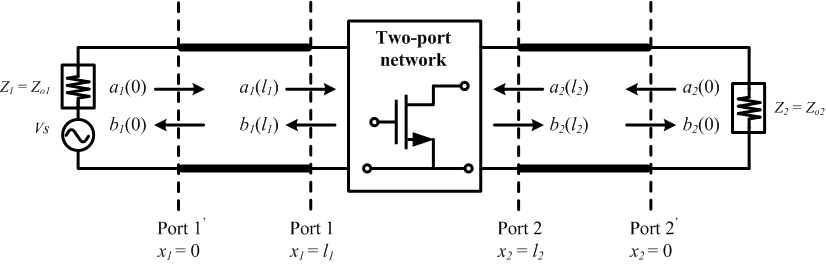Module 10.1 S-Parameters of Transistor
 |
 Freq (GHz) MagS11 (Magnitude) AngS11 (Phase Angle) MagS21 (Magnitude) AngS21 (Phase Angle) MagS12 (Magnitude) AngS12 (Phase Angle) MagS22 (Magnitude) AngS22 (Phase Angle)

Make a custom Sparameter.s2p file.
Step 1 : the Sparameter.s2p template file.
Step 2 : Open the Sparameter.s2p with a text editor.
Step 3 : Replace the value of the Freq., Mag., and Ang. with your device S parameters
Step 4 : Save the file and exit.
Step 5 : Browse and submit your new Sparameter.s2p file.

 Introduction:"Scattering matrix" [S]=[[S_(11),S_(12)],[S_(21),S_(22)]] a _1 (l_1) : "incident wave at port1" b _1 (l_1) : "reflected wave at port1" a _2 (l_2) : "incident wave at port2" b _2 (l_2) : "reflected wave at port2" S_12=| (b_1(l_1))/(a_2 (l_2))|_(a_1 (l_1 )=0)  "Reverse transmission coefficient with input properly terminated" S_11=| (b_1 (l_1))/(a_1 (l_1))|_(a_2 (l_2 )=0)  "Input reflection coefficient with output properly terminated" S_21=| (b_2 (l_2))/(a_1 (l_1))|_(a_2 (l_2 )=0)  "Forward transmission coefficient with output properly terminated" S_22=| (b_2 (l_2))/(a_2 (l_2))|_(a_1 (l_1 )=0)  "Output reflection coefficient with input properly terminated"